# 科普：String hashCode 方法为什么选择数字31作为乘子

+关注继续查看

## 2. 选择数字31的原因

public int hashCode() {
int h = hash;
if (h == 0 && value.length > 0) {
char val[] = value;

for (int i = 0; i < value.length; i++) {
h = 31 * h + val[i];
}
hash = h;
}
return h;
}

s*31^(n-1) + s*31^(n-2) + ... + s[n-1]

假设 n=3
i=0 -> h = 31 * 0 + val
i=1 -> h = 31 * (31 * 0 + val) + val
i=2 -> h = 31 * (31 * (31 * 0 + val) + val) + val
h = 31*31*31*0 + 31*31*val + 31*val + val
h = 31^(n-1)*val + 31^(n-2)*val + val

The value 31 was chosen because it is an odd prime. If it were even and the multiplication overflowed, information would be lost, as multiplication by 2 is equivalent to shifting. The advantage of using a prime is less clear, but it is traditional. A nice property of 31 is that the multiplication can be replaced by a shift and a subtraction for better performance: 31 * i == (i << 5) - i. Modern VMs do this sort of optimization automatically.

As Goodrich and Tamassia point out, If you take over 50,000 English words (formed as the union of the word lists provided in two variants of Unix), using the constants 31, 33, 37, 39, and 41 will produce less than 7 collisions in each case. Knowing this, it should come as no surprise that many Java implementations choose one of these constants.

## 3. 实验及数据可视化

### 3.1 哈希值冲突率计算

public static Integer hashCode(String str, Integer multiplier) {
int hash = 0;
for (int i = 0; i < str.length(); i++) {
hash = multiplier * hash + str.charAt(i);
}

return hash;
}

/**
* 计算 hash code 冲突率，顺便分析一下 hash code 最大值和最小值，并输出
* @param multiplier
* @param hashs
*/
public static void calculateConflictRate(Integer multiplier, List<Integer> hashs) {
Comparator<Integer> cp = (x, y) -> x > y ? 1 : (x < y ? -1 : 0);
int maxHash = hashs.stream().max(cp).get();
int minHash = hashs.stream().min(cp).get();

// 计算冲突数及冲突率
int uniqueHashNum = (int) hashs.stream().distinct().count();
int conflictNum = hashs.size() - uniqueHashNum;
double conflictRate = (conflictNum * 1.0) / hashs.size();

System.out.println(String.format("multiplier=%4d, minHash=%11d, maxHash=%10d, conflictNum=%6d, conflictRate=%.4f%%",
multiplier, minHash, maxHash, conflictNum, conflictRate * 100));
}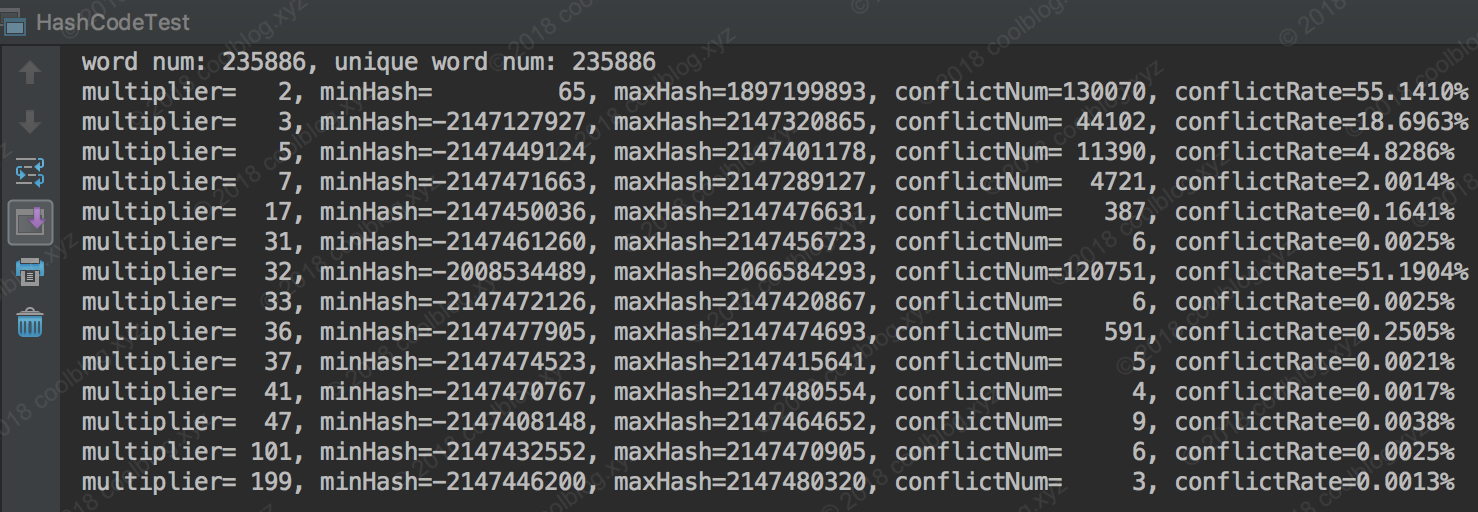### 3.2 哈希值分布可视化

 /**
* 将整个哈希空间等分成64份，统计每个空间内的哈希值数量
* @param hashs
*/
public static Map<Integer, Integer> partition(List<Integer> hashs) {
// step = 2^32 / 64 = 2^26
final int step = 67108864;
List<Integer> nums = new ArrayList<>();
Map<Integer, Integer> statistics = new LinkedHashMap<>();
int start = 0;
for (long i = Integer.MIN_VALUE; i <= Integer.MAX_VALUE; i += step) {
final long min = i;
final long max = min + step;
int num = (int) hashs.parallelStream()
.filter(x -> x >= min && x < max).count();

statistics.put(start++, num);
}

// 为了防止计算出错，这里验证一下
int hashNum = nums.stream().reduce((x, y) -> x + y).get();
assert hashNum == hashs.size();

return statistics;
}

0 -2147483648 -2080374784 32 0 67108864
1 -2080374784 -2013265920 33 67108864 134217728
2 -2013265920 -1946157056 34 134217728 201326592
3 -1946157056 -1879048192 35 201326592 268435456
4 -1879048192 -1811939328 36 268435456 335544320
5 -1811939328 -1744830464 37 335544320 402653184
6 -1744830464 -1677721600 38 402653184 469762048
7 -1677721600 -1610612736 39 469762048 536870912
8 -1610612736 -1543503872 40 536870912 603979776
9 -1543503872 -1476395008 41 603979776 671088640
10 -1476395008 -1409286144 42 671088640 738197504
11 -1409286144 -1342177280 43 738197504 805306368
12 -1342177280 -1275068416 44 805306368 872415232
13 -1275068416 -1207959552 45 872415232 939524096
14 -1207959552 -1140850688 46 939524096 1006632960
15 -1140850688 -1073741824 47 1006632960 1073741824
16 -1073741824 -1006632960 48 1073741824 1140850688
17 -1006632960 -939524096 49 1140850688 1207959552
18 -939524096 -872415232 50 1207959552 1275068416
19 -872415232 -805306368 51 1275068416 1342177280
20 -805306368 -738197504 52 1342177280 1409286144
21 -738197504 -671088640 53 1409286144 1476395008
22 -671088640 -603979776 54 1476395008 1543503872
23 -603979776 -536870912 55 1543503872 1610612736
24 -536870912 -469762048 56 1610612736 1677721600
25 -469762048 -402653184 57 1677721600 1744830464
26 -402653184 -335544320 58 1744830464 1811939328
27 -335544320 -268435456 59 1811939328 1879048192
28 -268435456 -201326592 60 1879048192 1946157056
29 -201326592 -134217728 61 1946157056 2013265920
30 -134217728 -67108864 62 2013265920 2080374784
31 -67108864 0 63 2080374784 2147483648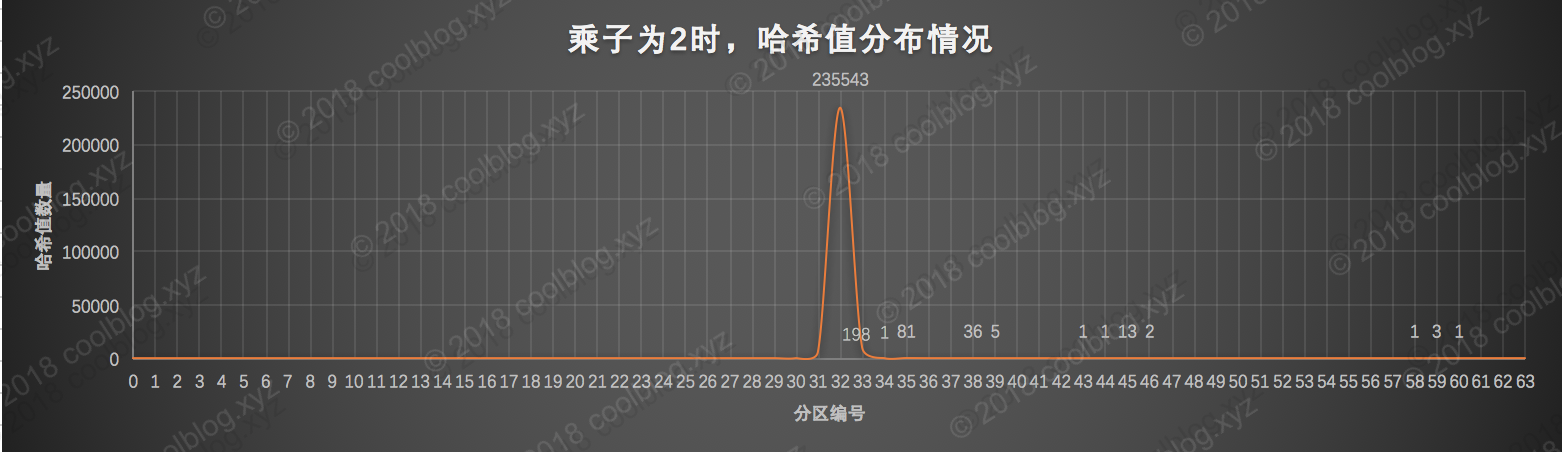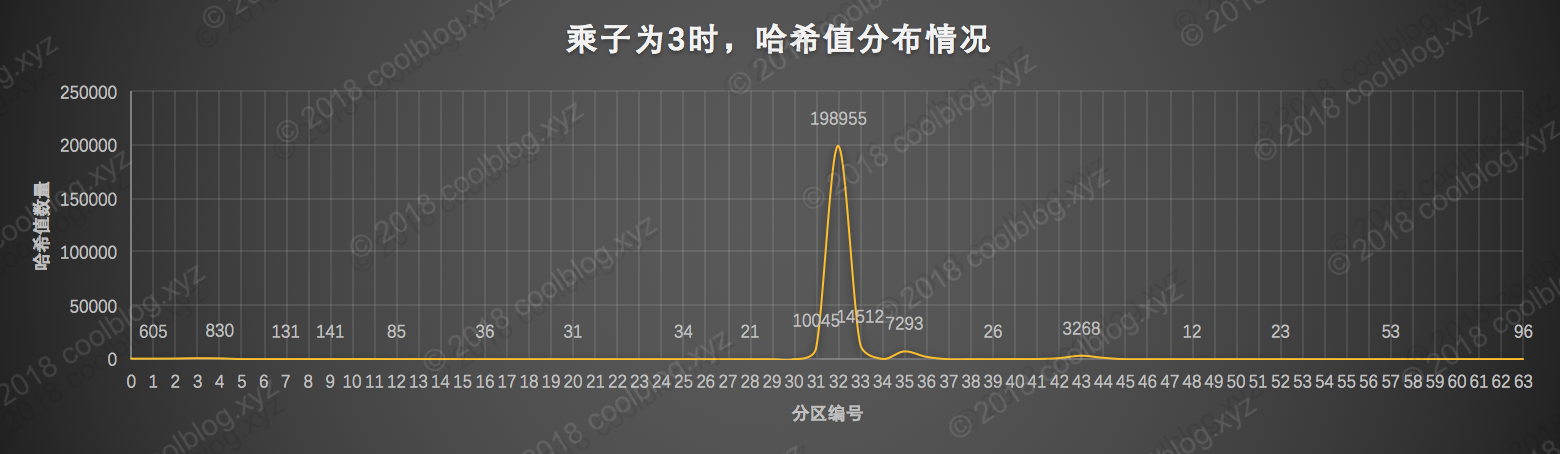3作为乘子时，算出的哈希值分布情况和2很像，只不过稍微好了那么一点点。从图中可以看出绝大部分的哈希值最终都落在了第32分区里，哈希值的分布性很差。这个也没啥用，拖出去枪毙5分钟吧。在看看数字17的情况怎么样：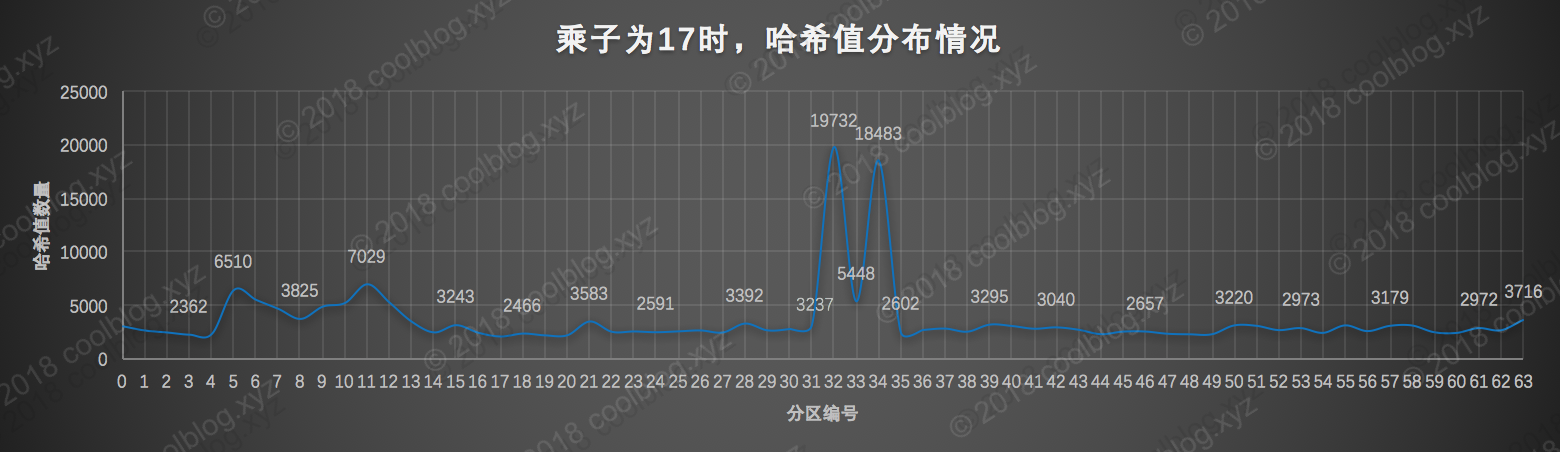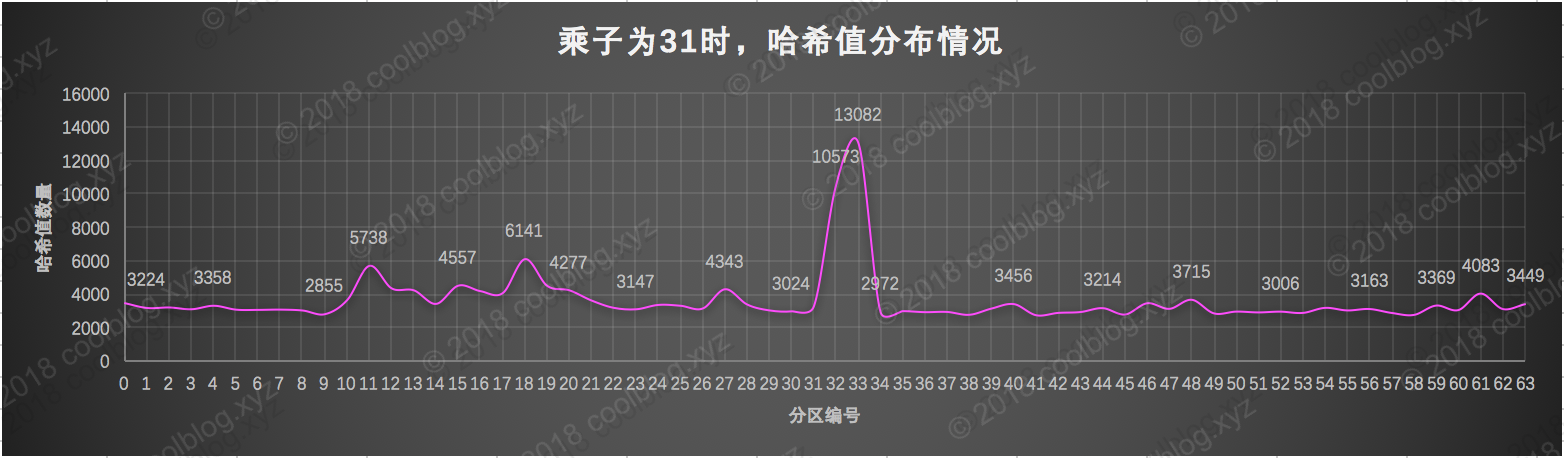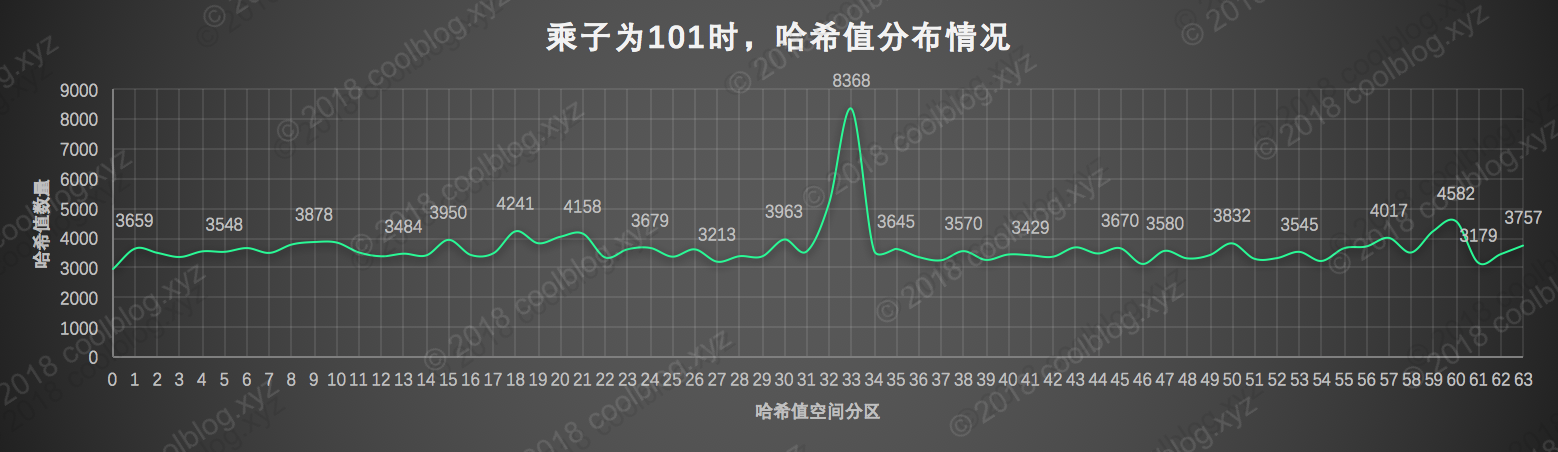## 4.写在最后Hystrix之外健壮微服务的新选择：Sentinel 发布首个生产版本
2649 01. 背景 某天，我在写代码的时候，无意中点开了 String hashCode 方法。然后大致看了一下 hashCode 的实现，发现并不是很复杂。但是我从源码中发现了一个奇怪的数字，也就是本文的主角31。
963 02050 0《Unity开发实战》——3.7节创建颜色选择对话框
1226 04478 0《STM32库开发实战指南：基于STM32F4》----2.4 选择目标板
1852 0jQuery.API源码深入剖析以及应用实现(3) － 选择器篇(上)

883 0911 01201 0《算法设计与分析》一一3.1　基于遍历的选择与查找

840 0

32

0

《SaaS模式云原生数据仓库应用场景实践》

《看见新力量：二》电子书